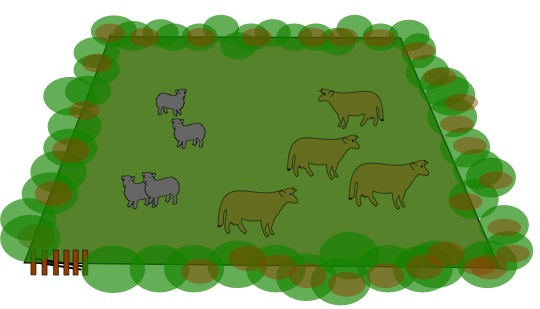#### You may also like### Exploring Wild & Wonderful Number Patterns

EWWNP means Exploring Wild and Wonderful Number Patterns Created by Yourself! Investigate what happens if we create number patterns using some simple rules.### Sending Cards

This challenge asks you to investigate the total number of cards that would be sent if four children send one to all three others. How many would be sent if there were five children? Six?### Dice and Spinner Numbers

If you had any number of ordinary dice, what are the possible ways of making their totals 6? What would the product of the dice be each time?

# Cows and Sheep

## Cows and SheepIn this field we could say, because of the number of animals there, that each cow can see 4 sheep and 3 (other) cows. This could be worded as follows "Each cow can see one more sheep than cows." There are obviously 4 sheep and 4 cows in the field as you see it.
But here are some questions about different fields in which you have to find out how many sheep and cows there are in each field.

In field number 1, each cow can see twice as many sheep as cows; each sheep can see the same number of sheep as cows, so how many cows and sheep are there?

In field number 2, each cow can see three times as many sheep as cows; each sheep can see the same number of sheep as cows, so how many cows and sheep are there?

In field number 3, each cow can see twice as many sheep as cows; each sheep can see one more sheep than cows, so how many cows and sheep are there?

In field number 4, each cow can see twice as many sheep as cows; each sheep can see two more sheep than cows, so how many cows and sheep are there?

In field number 5, each cow can see three times as many sheep as cows; each sheep can see twice as many sheep as cows, so how many cows and sheep are there?

### Why do this problem?

This problem challenges children to think in an organised way - the main aim is to help them develop a system or logical approach for tackling it.

### Possible approach

Begin by showing the group the picture in the problem. Rather than talking through the text underneath it, ask some questions about what they see, for example:
• What can you tell me about the number of sheep and cows in the picture?
• How many cows can each sheep see?
• How many cows can each cow see?
• How does the number of sheep a cow can see relate to the number of cows it can see?
Children might well say things like "each cow can see more sheep than cows", so ask them to be more exact. This may lead to statements like "each cow can see one more sheep than cows", or "each cow can see one less cow than sheep".

Try looking at the first field together. Simply read out what it says and ask the children to think about HOW they might find out the number of cows and sheep. Invite suggestions from the class. Choose a way to try which captures the idea of trial and improvement. If the children haven't had much experience of this, you might want to model a way for them as a start. So, focusing either on the sheep's view or the cows' view, try the smallest number that there could be to start with, increasing this one at a time, checking to see whether the results fit with the information at each stage. Emphasise that this way of approaching the problem, (trying the simplest case and then working up a step at a time) is a very good way of going about solving it as it means no possibilities are left out.

### Key questions

What shall we try first?
If there was just one cow, how many sheep would it see? How many cows would it see? Does this fit the clues?
If not, imagine there were two cows. How many sheep would each one see? How many cows would each one see? Does this fit the clues?